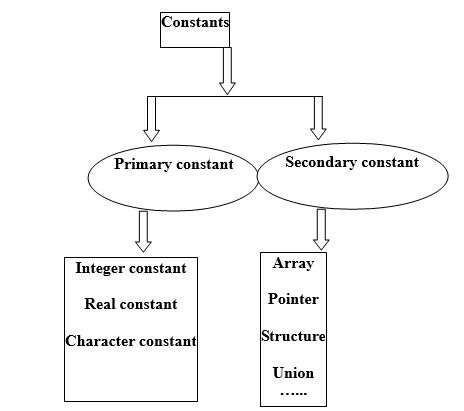# 04 - C Variables and Constants

This chapter is going to describe the nature and characteristics of C variables and constants.

Let us start with the main difference between constant and variable. A constant is a quantity which does not change upon execution and this can be stored at a location in memory of your machine. A variable is a name given to the location in memory where constant is stored and in contrast to constant it can change.

## 4.1 Types of ConstantsFigure C Constants

We are going to discuss primary constants in this chapter as we have separate chapters dealing with secondary constants. Let us have a brief idea about these constants:

### 1.Integer Constant:

• Integer constant is a whole number.
• Integer constant must have at least one digit.
• There should not be any decimal point.
• Integer constant may be a positive or negative number.
• Commas or blanks are not entertained within integer constants.
• The range of integer constants is -32,768 to +32767.
• Integer constant can be in turn divided into three sub categories and they are as follows:
• Decimal constant: Decimal constant can be defined as any combination of digits in the range of '0' to '9'. For example, +67, 120, -99, etc.
• Octal Constant: An octal constant can be defined as any combination of digits from '0' to '7' but there is a requirement that the number should have digit '0' as its prefix. For example, -065, 0666, etc.
• Hexadecimal Constant: Hexadecimal constant can be defined as any combination of digits in falling in range of digit '0' to digit '9' and letters from 'A' to 'F' or 'a' to 'f'. The requirement for this is that the number should be preceded by 0x or 0X. For example, 0XBC, 0x880, etc.

### 2.Real Constant:

• Constants with fractional part are called Floating point constants.
• Real constants are represented in two forms: Fractional form and Exponential form.
• Real constant should contain at least one digit.
• There has to be a decimal point.
• Commas or blanks are not entertained within floating point constants
• By default sign of the constant is positive.
• Example: 0.5, -0.99, etc.
• Exponential form of real constants is normally used when the number is too small or too large.
•  Exponential form has two parts: part appearing before 'e' is called mantissa and the part following 'e' is called the exponent. Hence mantissa part should be separated from the exponent part of the letter 'e'.
• Mantissa may have a positive or negative sign.
• By default, mantissa has positive sign.
• Exponent should have at least one digit which must be a positive or negative integer.
• Exponent also have a default positive sign.
• Real constants are expressed in a range of -3.4e38 to 3.4e38.
• Example: +3.2e-5, 4.4e+3

### 3.  Character Constant:

• Both inverted commas should point to left
• Maximum character constant length could be one character long
• Example, 'A', 'B', etc.
• Backslash constants/Escape sequence characters: This constant begins with a back slash followed by a character. Hence, they are also known as Backslash constants.
• A backslash followed by characters change the meaning of characters
• Non-printable back slash characters are: \a, \b, \t, \v, \n, \f, \r, and \0.
• String constants: Sequence of characters enclosed within pair of double quotes is called String constant.
• String constant ends with \0. \0 is called Null character.
• Example, “P”, “9”, etc.

## 4.2 Variables

Variable as the name suggests, it changes with execution. These are the variables which identify specific elements of the program. They are also called identifiers. Variable names are the names which are given to locations in the memory of development machine or computer where different constants are stored. These placeholders can hold an integer, real or character constant. Let us consider the following important points about variables:

• The name of a variable can be any combination of alphabets, digits or underscores.
• First character of the variable name must be an alphabet or an underscore.
• Commas or blanks are not allowed within variable name
• Except underscore, no special symbol is allowed in a variable name
• Variable names in lowercase are a good programming practice however, it is not mandatory
• Keywords should not be used as  variable names
• The C language is case sensitive, so int x and it X are two different integer variables
• Declaration of variable: In C language, all variables must be declared before using them. It reserves, the amount of memory required for these variables. Format of declaring variable is as follows:

Format- data_type name_of_variable semicolon

Example- int x;

• Assigning value to variable: Now we know that each variable represents a memory location where data is stored. Hence, each variable is usually associated with a value. This complete procedure of assigning value to variable is known as Assignment of values. It requires an “=” assignment operator to assign a value to variable.

Format- name_of_variable = value semicolon

Example- x=10;

We are concluding this chapter with this. Next chapter will introduce operators in the C language. Thank you.